【一猫汽车网】陕西西安，古称长安，先后有十三个王朝在此定都，是名副其实的帝王古都、千古名城，东风凯普特·第二届中国卡友节油挑战赛西北赛区复赛在这拉开战幕。秋风习习，让经历了十三个朝代洗礼的西安古城平添了一份苍凉；战鼓隆隆，西北的节油英豪们为节油大赛再添浓墨重彩！新旧的碰撞，正是今日西安的魅力所在，这是一场初出茅庐的新人与经验丰富的老将之间的对决。经过了一天的激战，卡友们的座次终于排定，来自西安赛区的年轻小将王辉以百公里5.050L的惊艳成绩夺冠，二度出征的老将全彦章及王驴娃分列二、三位，三名卡友将代表西北大区携手进军全国总决赛。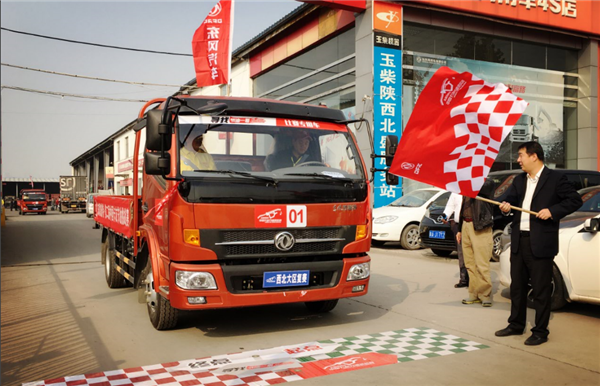在节油赛场上，老司机相对更容易摘得桂冠，几十年的驾车经验，让他们拥有纯熟的节油技巧，在比赛中更加游刃有余。然而，在西北赛区复赛的赛场上，最终夺得冠军的，却是一位驾龄只有四年的青年才俊——来自西安赛区的王辉。

今年只有26岁的王辉，在海选赛便是西安赛区的冠军得主。他性格温和、略带羞涩，在得知自己获得复赛第一名时，他第一时间将好消息发短信告知给家中妻子，脸上的笑容也明显轻松了许多。家中妻儿是王辉前进的动力和不变的牵挂。两年前，王辉辞掉在老家渭南县城体面的工作，要来西安拼闯一番，当时还是女友的妻子毅然选择支持他。现在王辉在西安为一家电动车经销商送货给下属代理商，工作时间长、强度大，而且需要随叫随到，但收入也比以前高了很多。能够给家人提供一个好的生活环境，王辉觉得再辛苦也值得：“趁年轻自己拼一把，让家里不至于为了金钱而发愁，再苦再累也会觉得甘甜了。”

虽然驾驶轻卡的时间并不长，但是王辉对于节油，却十分驾轻就熟。由于平时都是驾驶自己的东风多利卡挣钱养家，所以王辉在日常的驾驶运输中就非常有节油意识，比赛中他稳定发挥果然赛出了好成绩。比赛之余，王辉时不时翻看手机里妻子和儿子的照片，嘴角一直上扬着。他现在的目标是在西安买房，让妻子和孩子能有一个安稳温馨的家，以后孩子在大城市里成长也更有利。或许，在向梦想前行的路上，家人和责任就是他最强大的动力。这匹青春无敌的西北狼，是否能在决赛中问鼎？让我们拭目以待。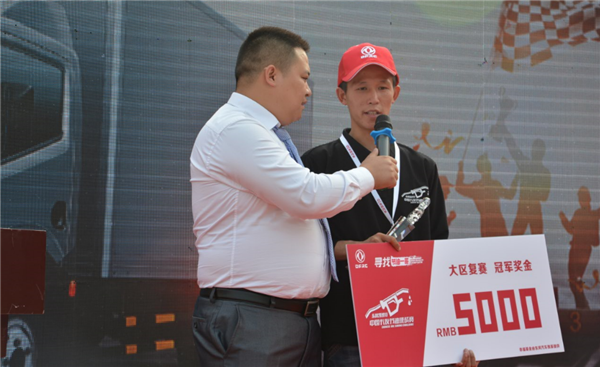能够杀入一次节油大赛总决赛，可能多少有些运气成分。可是连续两年杀入决赛，却绝对是节油实力的体现。在今年西北赛区复赛的颁奖台上，站在亚军位置的仍然是笑容憨厚的“老司机”——来自兰州赛区的全彦章师傅。

全彦章师傅其貌不扬，平常在市场上拉些散货，开车12年，已磨练出一手过硬的技术，尤其是节油技术在周围的卡友中都是极出色的。2015年东风汽车主办了第一届中国卡友节油挑战赛，全彦章过关斩将杀入了决赛并最终斩获全国第四名，这在朋友圈里引起了轰动，大家不但对他刮目相看，而且明显多了尊敬甚至崇拜。参加节油赛，全彦章不但收获了荣耀，也认识了许多朋友，赢得了友谊。“以前我都不怎么会用手机，因为节油赛我认识了全国各地的卡友兄弟，现在我们每天都在微信群里聊天，可热闹了。”在群里，全彦章和大家聊节油、聊生意、聊车况、聊生活，爱车之人，总是有说不完的话题。除了交了不少朋友，通过节油赛全师傅的环保节能意识、对规范驾驶的严格执行和追求都有了提升。现在的全彦章在每一次行车中像在比赛中那样严格要求自己，已形成了一种习惯，他也希望通过自己的好成绩让更多的卡友培养起节油意识。

作为东风轻卡的忠实用户，全师傅不仅对于东风轻型车的节油性赞不绝口，也肯定了东风轻型车的强大动力和稳定性能，他的日常工作车是一台风雨同行多年的东风多利卡。虽然这次没能比去年更进一步，西北称王，但是全师傅仍然非常开心自己能够杀入最终的决赛。他已经在去年的节油一哥微信群里向好兄弟们报喜：“大家加油，总决赛再相见！”我们非常期待，在今年的比赛中，这位憨厚的老司机可以再进一步，向全国节油一哥的宝座进发。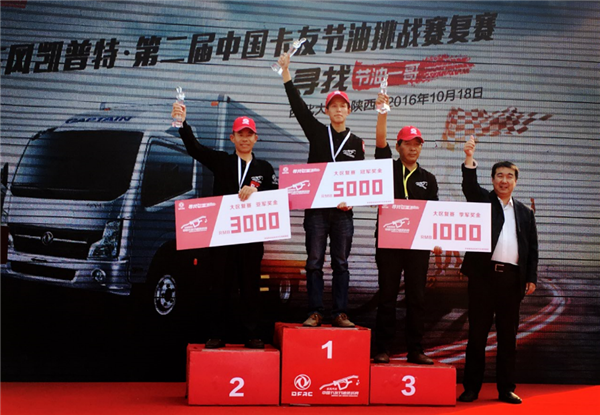以成绩领先第四名0.007L的微小优势晋级总决赛，王驴娃无疑是幸运的！然而，幸运依旧是实力的体现，毕竟在高手云集的大区复赛上，能够杀入前三名绝不仅仅靠运气。多年的轻卡驾驶经验，让王师傅对于自己的节油技巧非常自信；而作为东风凯普特的老用户，他对于比赛中使用的凯普特车型也是驾轻就熟，取得好成绩也在意料之中。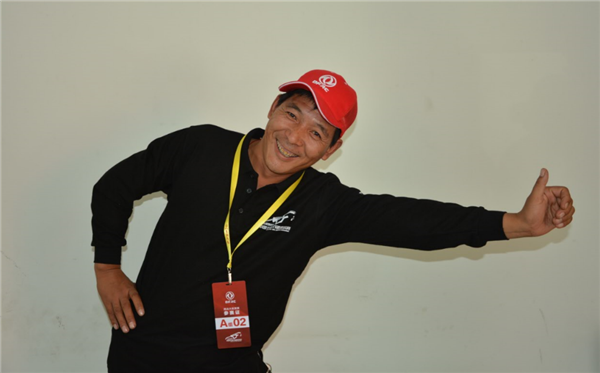王驴娃是个地道的山西农村汉子，除了耿直，还十分诙谐，总能语出惊人，引得大伙儿乐开花。王师傅的老家山西运城是有名的水果产地，他从乡亲们手里收购时令瓜果，运到城里卖给商贩。由于爱笑随性、与人为善，王师傅积累了很多忠实客户，大伙儿都愿意跟他打交道，商贩们也成为他的忠实客户。现在王驴娃手头仅固定大客户就有数十个，水果旺季他还需要专门雇人帮他运送水果。年初，在朋友的介绍下，王驴娃新入手了一辆东风轻卡，而这辆东风轻卡的优质性能也很快让王驴娃成为东风的忠实客户：“皮实，动力好，关键是东风的服务有保证。现在这辆比我以前用的车顺手，明年我把那辆车也要换成东风轻卡。”

比起出色的节油战绩，王师傅乐观爱笑的性格，更加让人印象深刻。这位爱笑的卡友，不仅在节油赛现场与其他卡友们谈天说地，成为现场的开心果，也积极热情地参加着现场的趣味小游戏，享受着辛苦的运输生活中难得的轻松。俗话说，爱笑的人运气都不会差，我们也非常期待在决赛中再次见到这位乐观的卡友师傅。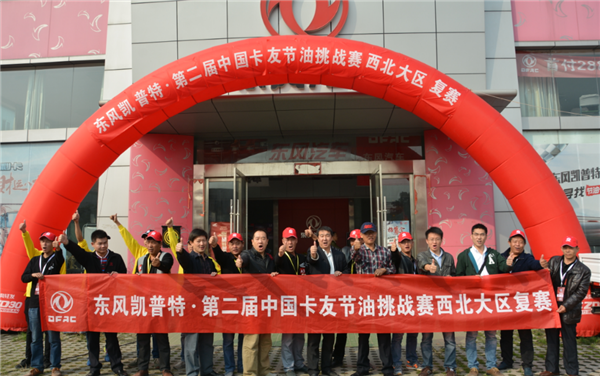在古城西安，我们看到了新一代卡友的奋勇登顶，也见识到了老一代卡友的宝刀未老，更看到了卡友这个群体的热情与活力。新老卡友们的对决，展示出轻卡这个行业的蓬勃发展，这正是节油赛的魅力，也是卡友这个群体的魅力，他们用平凡诠释着属于自己的精彩。随着节油大赛的不断推进，卡友们乐观，积极的生活态度、用于挑战自我的风采会得到越来越全面而鲜明的展现，真心期待他们在全国总决赛再接再厉，再创佳绩！

##### 相关资讯

|分享

•已阅！握爪
•我手滑为你点赞
•128个赞！
•不明觉厉
•阅后既醉
•有钱！任性
•照片太美，我不敢看
•干货！杠杠的
•高大上
•膜拜中！
•请接受我的膝盖
•猫哥/猫妹么么哒
•神吐槽
•我只笑笑不说话
•我想静静
•窒息
##### 资讯相关车系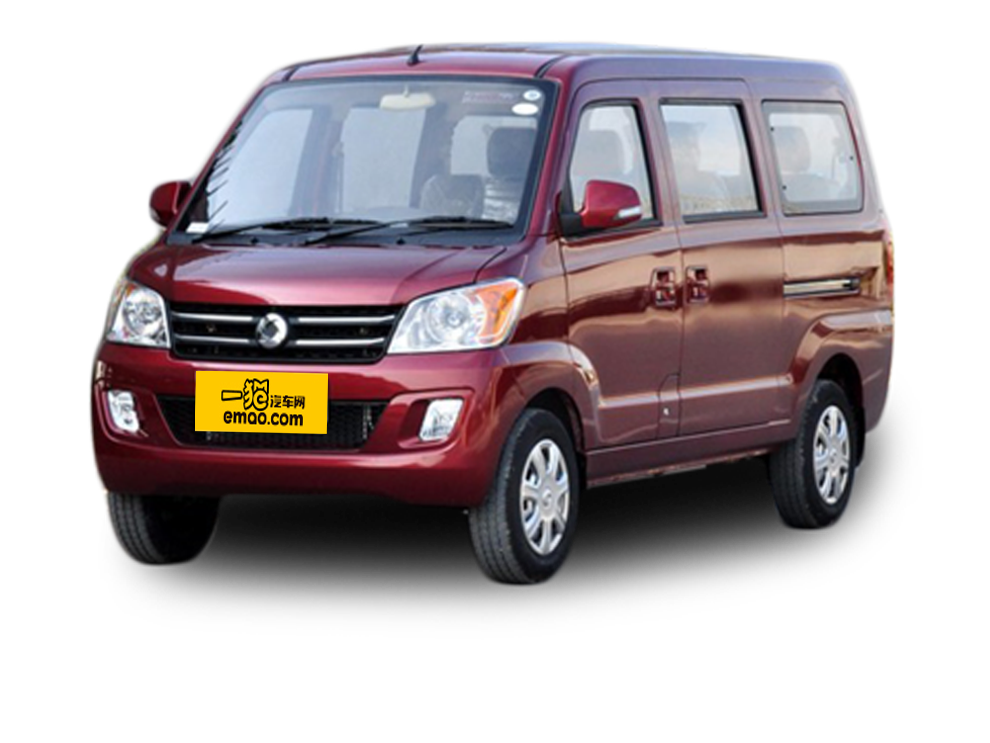##### 热度排行• 阿斯顿·马丁
• 奥迪
• 阿尔法罗密欧
• ALPINA

• 巴博斯
• 宝骏
• 宝马
• 保时捷
• 北汽制造
• 奔驰
• 奔腾
• 本田
• 比亚迪
• 标致
• 别克
• 宾利
• 布加迪
• 北汽威旺
• 北京
• 北汽绅宝
• 北汽幻速
• 北汽新能源
• 宝沃
• 比速汽车
• 北汽道达

• 昌河
• 长安
• 长城
• 长安商用
• 成功汽车
• 长江EV
• 长安轻车型

• 大众
• 道奇
• 东风
• 东风风神
• 东风小康
• 东南
• DS
• 东风风行
• 东风风度
• 东风风光

• 法拉利
• 菲亚特
• 丰田
• 福迪
• 福特
• 福田汽车
• 福汽启腾
• 风诺

• GMC
• 广汽传祺
• 广汽吉奥
• 观致

• 哈飞
• 海格
• 海马
• 华泰
• 黄海
• 恒天
• 红旗
• 哈弗
• 华颂
• 华凯
• 华泰新能源
• 汉腾汽车

• Jeep
• 江淮
• 江铃
• 捷豹
• 金杯
• 九龙
• 吉利汽车
• 金旅
• 金龙
• 江铃集团轻汽
• 江铃集团新能源
• 君马汽车
• 捷途

• 开瑞
• 凯迪拉克
• 科尼赛克
• 克莱斯勒
• KTM
• 卡威
• 凯翼
• 康迪
• 康迪电动汽车集团

• 猎豹汽车
• 兰博基尼
• 劳斯莱斯
• 雷克萨斯
• 雷诺
• 理念
• 力帆
• 莲花汽车
• 林肯
• 铃木
• 陆风
• 路虎
• 路特斯
• 领志
• 领克
• 零跑汽车

• MG
• MINI
• 马自达
• 玛莎拉蒂
• 迈凯伦
• 摩根

• 纳智捷

• 讴歌
• 欧宝
• 欧朗
• 欧拉
• 欧尚汽车

• 帕加尼

• 奇瑞
• 启辰
• 起亚
• 前途
• 庆铃汽车

• 日产
• 荣威
• 瑞麒汽车
• 如虎
• 瑞驰

• 上汽大通
• smart
• 三菱
• 双环
• 双龙
• 斯巴鲁
• 斯柯达
• 萨博
• 思铭
• 赛麟
• SWM斯威汽车

• TESLA
• 泰卡特
• 腾势

• 威麟
• 威兹曼
• 沃尔沃
• 五菱汽车
• 五十铃
• 潍柴英致
• WEY
• 蔚来
• 威马汽车

• 现代
• 雪佛兰
• 雪铁龙
• 西雅特
• 新特汽车
• 小鹏汽车
• 新宝骏

• 野马汽车
• 一汽
• 依维柯
• 英菲尼迪
• 永源
• 驭胜

• 中华
• 中兴
• 众泰
• 知豆
• 之诺
• 正道汽车
• A
• B
• C
• D
• E
• F
• G
• H
• I
• J
• K
• L
• M
• N
• O
• P
• Q
• R
• S
• T
• U
• V
• W
• X
• Y
• Z

• 阿斯顿·马丁
• 奥迪
• 阿尔法罗密欧
• ALPINA

• 巴博斯
• 宝骏
• 宝马
• 保时捷
• 北汽制造
• 奔驰
• 奔腾
• 本田
• 比亚迪
• 标致
• 别克
• 宾利
• 布加迪
• 北汽威旺
• 北京
• 北汽绅宝
• 北汽幻速
• 北汽新能源
• 宝沃
• 比速汽车
• 北汽道达

• 昌河
• 长安
• 长城
• 长安商用
• 成功汽车
• 长江EV
• 长安轻车型

• 大众
• 道奇
• 东风
• 东风风神
• 东风小康
• 东南
• DS
• 东风风行
• 东风风度
• 东风风光

• 法拉利
• 菲亚特
• 丰田
• 福迪
• 福特
• 福田汽车
• 福汽启腾
• 风诺

• GMC
• 广汽传祺
• 广汽吉奥
• 观致

• 哈飞
• 海格
• 海马
• 华泰
• 黄海
• 恒天
• 红旗
• 哈弗
• 华颂
• 华凯
• 华泰新能源
• 汉腾汽车

• Jeep
• 江淮
• 江铃
• 捷豹
• 金杯
• 九龙
• 吉利汽车
• 金旅
• 金龙
• 江铃集团轻汽
• 江铃集团新能源
• 君马汽车
• 捷途

• 开瑞
• 凯迪拉克
• 科尼赛克
• 克莱斯勒
• KTM
• 卡威
• 凯翼
• 康迪
• 康迪电动汽车集团

• 猎豹汽车
• 兰博基尼
• 劳斯莱斯
• 雷克萨斯
• 雷诺
• 理念
• 力帆
• 莲花汽车
• 林肯
• 铃木
• 陆风
• 路虎
• 路特斯
• 领志
• 领克
• 零跑汽车

• MG
• MINI
• 马自达
• 玛莎拉蒂
• 迈凯伦
• 摩根

• 纳智捷

• 讴歌
• 欧宝
• 欧朗
• 欧拉
• 欧尚汽车

• 帕加尼

• 奇瑞
• 启辰
• 起亚
• 前途
• 庆铃汽车

• 日产
• 荣威
• 瑞麒汽车
• 如虎
• 瑞驰

• 上汽大通
• smart
• 三菱
• 双环
• 双龙
• 斯巴鲁
• 斯柯达
• 萨博
• 思铭
• 赛麟
• SWM斯威汽车

• TESLA
• 泰卡特
• 腾势

• 威麟
• 威兹曼
• 沃尔沃
• 五菱汽车
• 五十铃
• 潍柴英致
• WEY
• 蔚来
• 威马汽车

• 现代
• 雪佛兰
• 雪铁龙
• 西雅特
• 新特汽车
• 小鹏汽车
• 新宝骏

• 野马汽车
• 一汽
• 依维柯
• 英菲尼迪
• 永源
• 驭胜

• 中华
• 中兴
• 众泰
• 知豆
• 之诺
• 正道汽车
• A
• B
• C
• D
• E
• F
• G
• H
• I
• J
• K
• L
• M
• N
• O
• P
• Q
• R
• S
• T
• U
• V
• W
• X
• Y
• Z

• 阿斯顿·马丁
• 奥迪
• 阿尔法罗密欧
• ALPINA

• 巴博斯
• 宝骏
• 宝马
• 保时捷
• 北汽制造
• 奔驰
• 奔腾
• 本田
• 比亚迪
• 标致
• 别克
• 宾利
• 布加迪
• 北汽威旺
• 北京
• 北汽绅宝
• 北汽幻速
• 北汽新能源
• 宝沃
• 比速汽车
• 北汽道达

• 昌河
• 长安
• 长城
• 长安商用
• 成功汽车
• 长江EV
• 长安轻车型

• 大众
• 道奇
• 东风
• 东风风神
• 东风小康
• 东南
• DS
• 东风风行
• 东风风度
• 东风风光

• 法拉利
• 菲亚特
• 丰田
• 福迪
• 福特
• 福田汽车
• 福汽启腾
• 风诺

• GMC
• 广汽传祺
• 广汽吉奥
• 观致

• 哈飞
• 海格
• 海马
• 华泰
• 黄海
• 恒天
• 红旗
• 哈弗
• 华颂
• 华凯
• 华泰新能源
• 汉腾汽车

• Jeep
• 江淮
• 江铃
• 捷豹
• 金杯
• 九龙
• 吉利汽车
• 金旅
• 金龙
• 江铃集团轻汽
• 江铃集团新能源
• 君马汽车
• 捷途

• 开瑞
• 凯迪拉克
• 科尼赛克
• 克莱斯勒
• KTM
• 卡威
• 凯翼
• 康迪
• 康迪电动汽车集团

• 猎豹汽车
• 兰博基尼
• 劳斯莱斯
• 雷克萨斯
• 雷诺
• 理念
• 力帆
• 莲花汽车
• 林肯
• 铃木
• 陆风
• 路虎
• 路特斯
• 领志
• 领克
• 零跑汽车

• MG
• MINI
• 马自达
• 玛莎拉蒂
• 迈凯伦
• 摩根

• 纳智捷

• 讴歌
• 欧宝
• 欧朗
• 欧拉
• 欧尚汽车

• 帕加尼

• 奇瑞
• 启辰
• 起亚
• 前途
• 庆铃汽车

• 日产
• 荣威
• 瑞麒汽车
• 如虎
• 瑞驰

• 上汽大通
• smart
• 三菱
• 双环
• 双龙
• 斯巴鲁
• 斯柯达
• 萨博
• 思铭
• 赛麟
• SWM斯威汽车

• TESLA
• 泰卡特
• 腾势

• 威麟
• 威兹曼
• 沃尔沃
• 五菱汽车
• 五十铃
• 潍柴英致
• WEY
• 蔚来
• 威马汽车

• 现代
• 雪佛兰
• 雪铁龙
• 西雅特
• 新特汽车
• 小鹏汽车
• 新宝骏

• 野马汽车
• 一汽
• 依维柯
• 英菲尼迪
• 永源
• 驭胜

• 中华
• 中兴
• 众泰
• 知豆
• 之诺
• 正道汽车
• A
• B
• C
• D
• E
• F
• G
• H
• I
• J
• K
• L
• M
• N
• O
• P
• Q
• R
• S
• T
• U
• V
• W
• X
• Y
• Z